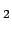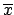Next: Indirect measurements Up: Evaluation of uncertainty: general Previous: Evaluation of uncertainty: general   Contents

## Direct measurement in the absence of systematic errors

The first step consists in evaluating the uncertainty on a quantity measured directly. The most common likelihoods which describe the observed values are the Gaussian, the binomial and the Poisson distributions.
Gaussian:
This is the well-known case of `normally' distributed errors. For simplicity, we will only considerindependent of(constant r.m.s. error within the range of measurability), but there is no difficulty of principle in treating the general case. The following cases will be analysed:
Binomial:
This distribution is important for efficiencies and, in the general case, for making inferences on unknown proportions. The cases considered include (see Section):
• general case with flat prior leading to the recursive Laplace formula (the problem solved originally by Bayes);
• limit to normality;
• combinations of different datasets coming from the same proportion;
• upper and lower limits when the efficiency is 0 or 1;
• comparison with Poisson approximation.
Poisson:
The cases of counting experiments here considered2.15 are (see Section):Next: Indirect measurements Up: Evaluation of uncertainty: general Previous: Evaluation of uncertainty: general   Contents
Giulio D'Agostini 2003-05-15